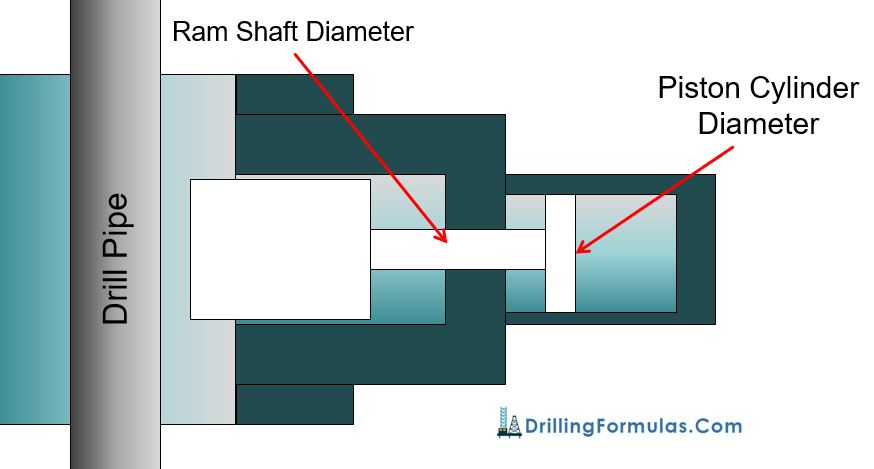## What is Closing Ratio in Blow Out Preventer (BOP)?People asked me about what the closing ratio is and what it tells us. Closing ratio is defined as the cross sectional area of the ram piston (cylinder) divided by the cross sectional area of the ram shaft. The closing ratio is used to determine Ram closing pressure which will overcome wellbore pressure acting to Ram body. The closing ratio formula is below;

### Closing Ratio = Ram Piston Cylinder Area ÷ Ram Shaft Area

Before going into the detailed calculation, we would like to show you where the cylinder and the ram shaft are in BOP. In Figure 1 (Shaffer SL-Ram BOP), the yellow shaded parts demonstrate these two areas which will be used to calculate the closing ratio.Figure 1 – Shaffer SL-Ram BOP

Detailed Formulas are as follows;

Ram Piston Cylinder Area  (square inch) = ( π× Ram Piston Cylinder Diameter) ÷ 4

Ram Shaft Area  (square inch) = ( π× Ram Shaft Diameter) ÷ 4

Closing Ratio = Ram Piston Cylinder Area ÷ Ram Shaft AreaFigure 2 – Diagram of Closing Ratio Calculation